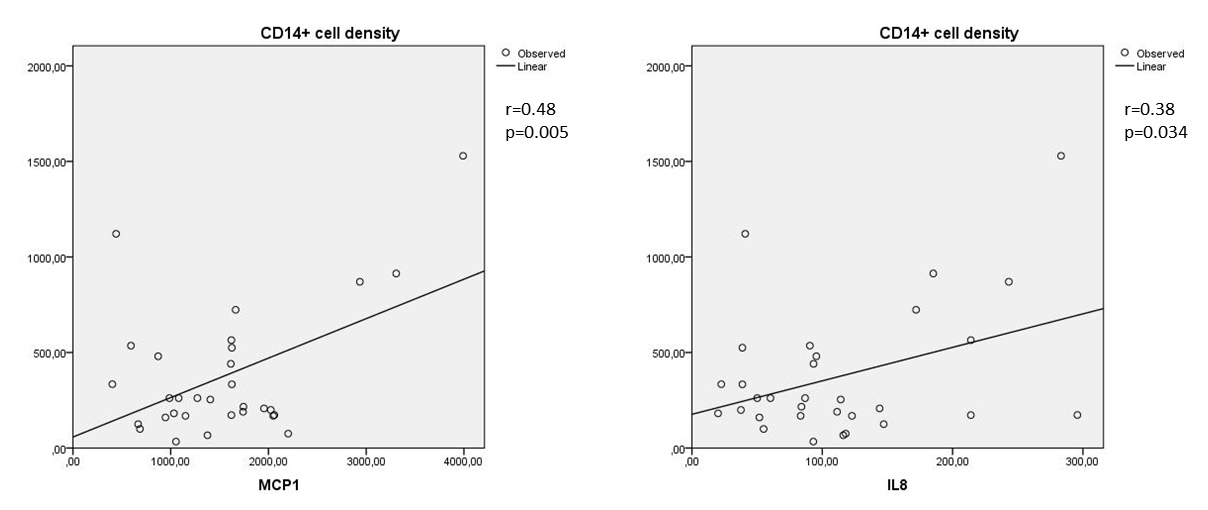Figure 3 of Urbančič, Mol Vis 2020; 26:472-482.

Figure 3. Correlation between MCP-1 and the numerical areal densities of monocytes/macrophages and between IL-8 and the numerical areal densities of monocytes/macrophages (Spearman's coefficent r). Left: correlation between MCP-1 and the numerical areal density of CD14+ cells (r = 0.48; p = 0.005); right: correlation between IL-8 and the numerical areal density of CD14+ cells (r = 0.38; p = 0.034). Numerical areal density, NA.